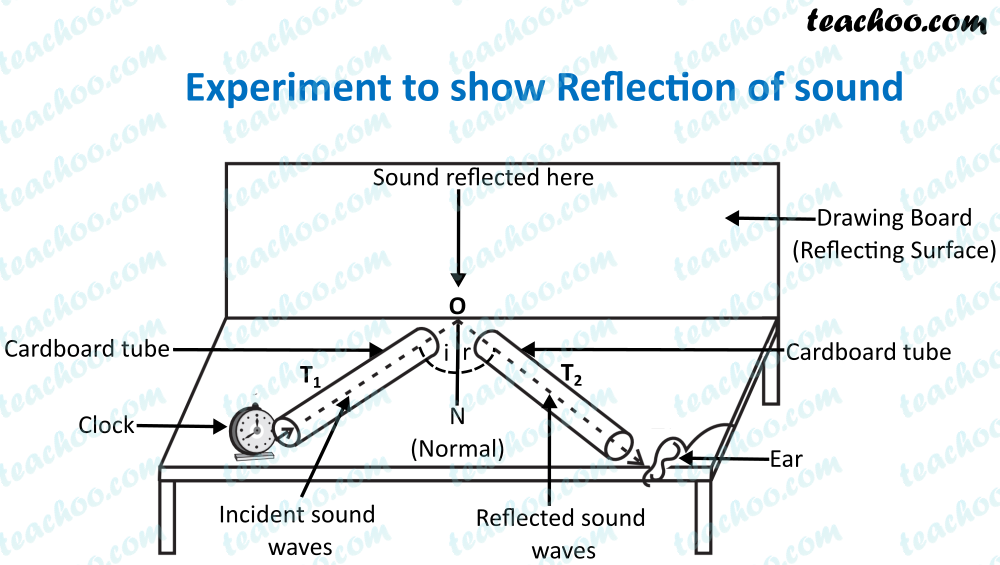NCERT Questions

Class 9
Chapter 12 Class 9 - Sound

## Does sound follow the same laws of reflection as light does? Explain.

Yes, sound follows the same laws of reflection as light.

Thus, in a sound wave

1. Incident Sound Wave,Reflected Sound Wave and Normal Sound Wave all lie on same plane
2. Angle of Incidence of Sound is equal to Angle of Reflection  of Sound

This can be proved by experiment

Experiment to Show Reflection of SoundMake 2 pipes of Chart Paper

Arrange them on Table near wall

Keep a clock near one of pipes

Try to hear from other pipe

Adjust position of second pipe till sound is largest

We draw a line perpendicular to point where 2 pipes meet

This is normal line

We Measure the angle at this place

At this position ,we observe that

Angle of Incidence of Sound is equal to Angle of Reflection  of Sound

Also, Incident Sound Wave, Reflected Sound Wave and Normal Sound Wave all lie on same plane (which is the table in this case)

Note : If it is a 2 mark question, write explanation but don’t write acitivity. If it is a 4 or 5 mark question, write explanation and activity both

Learn in your speed, with individual attention - Teachoo Maths 1-on-1 Class